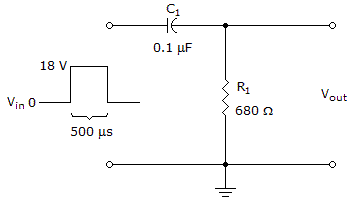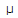# Electronics - Time Response of Reactive Circuits - Discussion

### Discussion :: Time Response of Reactive Circuits - Filling the Blanks (Q.No.5)

5.After the rising edge of the input pulse occurs, it takes ______ for the voltage across the resistor to decrease to zero in the given circuit.

 [A]. 0 s [B]. 68s [C]. 340s [D]. 500s

Explanation:

No answer description available for this question.

 Kiran V said: (Dec 22, 2016) T =RC, T = 680 * 0.1 * 10^-6, T = 68 u sec. The voltage across the resistor to decrease to zero in the given circuit, it needs 5 Time. Constants. 5T = 5 RC = 5 * 68 * 10^-6, 5T = 340 u sec.